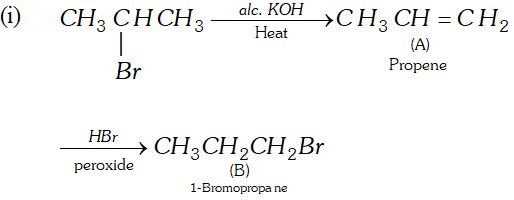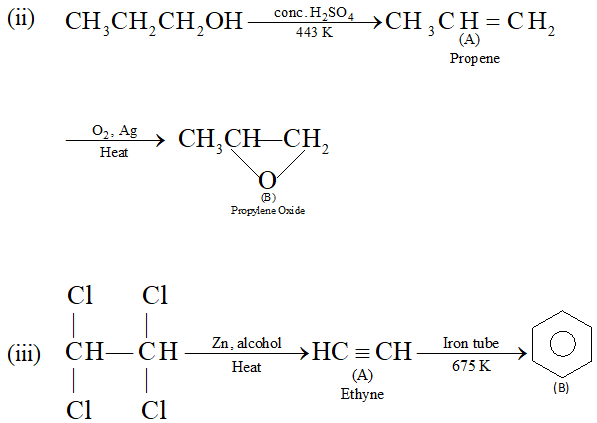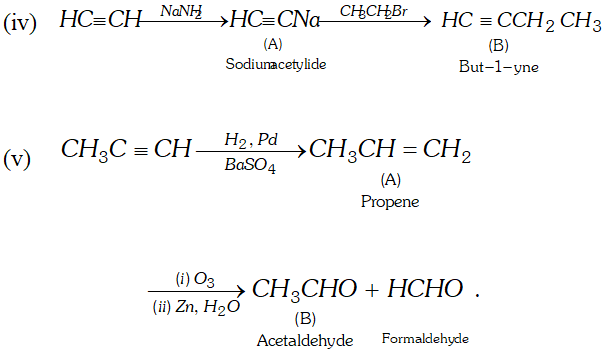Most Affordable JEE | NEET | 8,9,10 Preparation by Kota's Top IITian Doctor Faculties

# Aliphatic Hydrocarbon | Question Bank for Class 12 Chemistry`
Q. What are the natural sources of hydrocarbons ?
Ans. Coal and petroleum are two natural sources of hydrocarbons.
Q. What is petroleum ?
Ans. Petroleum is a complex mixture of solid, liquid and gaseous hydrocarbons.
Q. What is the main constituent of LPG ?
Ans. LPG mainly contains butane and Isobutane.
Q. Give a scale for measuring the quality of gasoline.
Ans. Octane number.
Q. Arrange the following in order of increasing volatility : gasoline, kerosene oil and diesel.
Ans. Diesel < kerosene oil < gasoline.
Q. Name the compound that is added to LPG to detect its leakage.
Ans. The compound added to LPG to detect its leakage is ethyl mercaptan $\left(C_{2} H_{5} S H\right)$
Q. Name the product obtained by the fractional distillation of petroleum which is used as a furnace fuel in metallurgical operations.
Ans. Fuel oil is used as furnace fuel in metallurgical operations.
Q. Define knocking.
Ans. A sharp metallic rattling sound produced in an internal combustion engine, is called knocking.
Q. What is octane number of fuel which contains $95 \%$ iso-octaneand $5 \%$ n-heptane?
Ans. Octane number is 95.
Q. What is meant by anti-knocking compound ? Give its example.
Ans. - Those compounds which reduces knocking and help in smooth combustion of gasoline are called antiknocking compounds, e.g. tetra ethyl lead $\left(C_{2} H_{5}\right)_{4} P b$
Q. Why is dipole moment of trans-1, 2 dichloroethene zero ?
Ans. It is because individual dipoles are equal and opposite such that net dipole moment is zero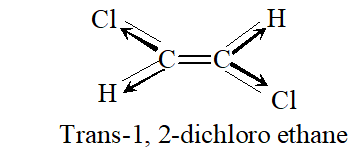Q. Why alkanes and cycloalkanes are called paraffins ?
Ans. They are called paraffins because under normal conditions alkanes are inert towards common reagents [In Latin, Parum = little, affinis =affinity]
Q. Why eclipsed and staggered forms of ethane cannot be isolated at room temperature ?
Ans. It is because the difference in their energy is less.
Q. What is Lindlar’s catalyst ? Give its use.
Ans. Lindlar's catalyst is $P d / B a S O_{4} .$ It is used to convert Alkynes to alkenes with the help of $H_{2}$
Q. Convert ethylene to ethane.
Ans. $\mathrm{CH}_{2}=\mathrm{CH}_{2}+\mathrm{H}_{2} \frac{\mathrm{Ni}}{573 \mathrm{K}} \mathrm{CH}_{3}-\mathrm{CH}_{3}$
Q. Geometrical isomers are not possible in alkynes why ?
Ans. Due to their straight linear geometry.
Q. Ethyne treated with ozone followed by treatment with zinc and water, leads to formation of compound ‘X’. What is X ?
Ans. X is glyoxal, i.e., ethane 1, 2-dial.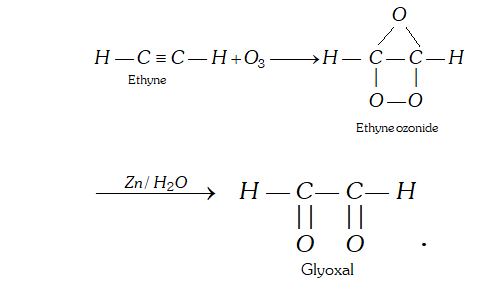Q. Write chemical equation for the reaction when ethyne is passed through acetic acid in the presence of $\mathrm{Hg}^{+}$.
Ans. In the presence of $H g^{+},$ ethyne adds acetic acid to give vinyl ester.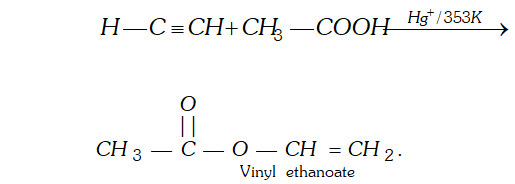Q. What are arenes ? Give general formula of monocyclic arenes.
Ans. - Arenes are aromatic hy drocarbons. $C_{n} H_{2 n-6}$ is general formula for monocyclic arenes.
Q. What is trade name of benzene hexachloride ?
Ans. Gammaxene.
Q. What is electrophile in sulphonation ?
Ans. $\mathrm{SO}_{3} \mathrm{H}^{+}$
Q. In presence of conc. $H_{2} S O_{4},$ conc. $H N O_{3}$ acts as acid or base during nitration of benzene?
Ans. It acts as a base.
Q. Write down the products of ozonolysis of $1,2$ dimethylbenzene as cyclohexa- $1,3,5$ -triene.
Ans. With ozone $o$ -xylene forms triozonide that supports the structure of benzene as cyclohexa- $1,3,5$ triene.
Q. How is petroleum refined using technique of fractional distillation ?
Ans. The crude oil is washed with acidic or basic solution depending upon whether the impurities are basic or acidic in nature. The washed oil is then subjected to fractional distillation by heating it to about $723 K$ and allowing the vapours to pass through a fractionating column. The crude oil is separated into various fractions.
Q. Give the full form of CNG and state its composition.
Ans. CNG is compressed natural gas. It mainly consists of methane, $(85$ to $95 \%$ ). Ethane and propane are also present in small quantities. Natural gas is formed in the earth crust by the decomposition of vegetable-matter lying under water by the action of anaerobic bacteria so mostly it is found along with petroleum deposits.
Q. Discuss the stability of conformers of ethane.
Ans. The staggered conformer of ethane is more stable than eclipsed conformer, because in staggered conformer, the H-atoms are far apart. So, the repulsion is lesser than in eclipsed conformation. The difference in the energy content of staggered and eclipsed conformation is $12.5 \mathrm{kJ} \mathrm{mol}^{-1}$ The order of stability of conformers is in the following order: Staggered $>$ gauche (skew) $>$ eclipsed.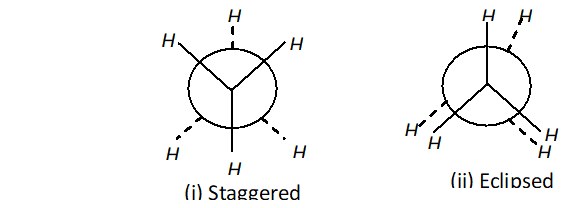Q. Classify the following as Z or E isomers :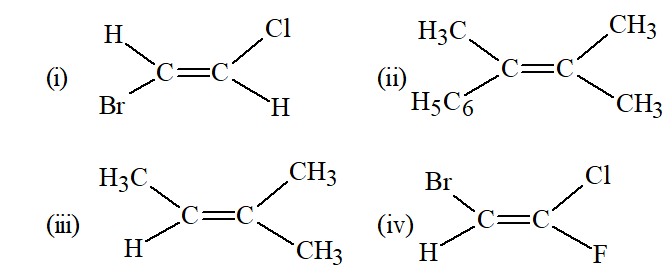Ans. (i) E (ii) E (iii) E (iv) Z
Q. What is cracking ? Describe the methods to complete this process.
Ans. Cracking : "The process of breaking down of hydrocarbons of high molecular mass and higher boiling points into smaller hydrocarbons of low molecular mass and lower boiling points, is termed as cracking. For Example: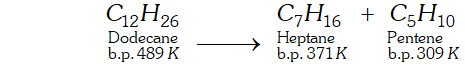Types of cracking : The process of cracking is of two types: (i) Thermal cracking. (ii) Catalytic cracking. (i) Thermal cracking: When cracking is carried at high temperature, it is called thermal cracking. (ii) Catalytic Cracking: When cracking is carried at low temperature in the presence of a catalyst such as alumina or silica, is called catalytic cracking.
Q. In the alkane $C H_{3}-C H_{2} C\left(C H_{3}\right)_{2} C H_{2} C H\left(C H_{3}\right)_{2},$ identify $1^{\circ}, 2^{\circ}, 3^{\circ}$ and $4^{\circ}$ carbon atoms and give the total number of $H$ atoms bonded to each one of these.
Ans.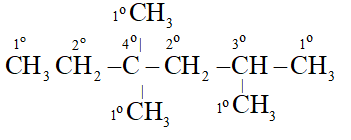Each $1^{\circ}$ Carbon is attached to 3 hydrogen, Each $2^{\circ}$ carbon is attached to 2 hydrogen atoms. Each tertiary 'C' atom is bonded to one and quaternary $^{\epsilon} \mathrm{C}^{\prime}$ is not attached to any hydrogen atom. All five Carbon atoms are attached to total 15 hydrogen atoms. TwoCarbon atoms are attached to total 4 hydrogen atoms. OneCarbon atom is attached to total one hydrogen atom OneCarbon atom is not attached to any hydrogen atom.
Q. Draw Newman projection formulae of the two staggered Gauch conformations of n-butane. [NCERT]
Ans.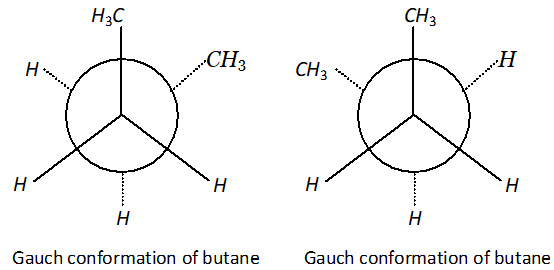Q. Between the two conformational isomers of cyclohexane, i.e., chair and boat forms, which one is more stable ? [NCERT]
Ans. Chair form is more stable than boat form.
Q. Addition of to propene yields bromo-2-propane, while in presence of benzoyl peroxide the same reaction yields 1-bromopropane. Explain and give mechanism. [NCERT]
Ans.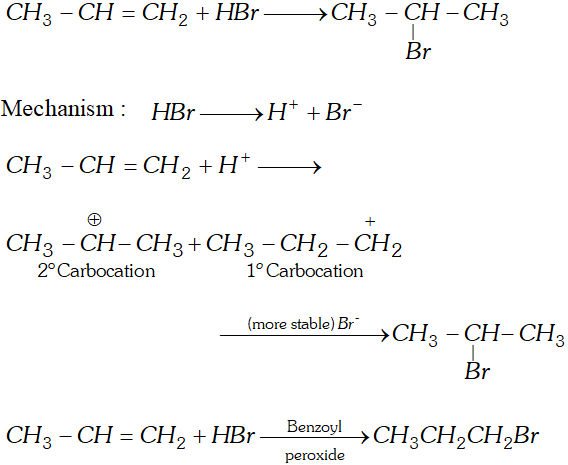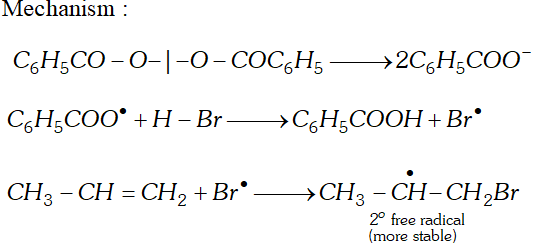Q. Why propane has only one eclipsed conformation while butane has three ? Explain and give diagrams.
Ans. Propane has only one eclipsed conformer Butane has three eclipsed forms due to changes in dihedral angles.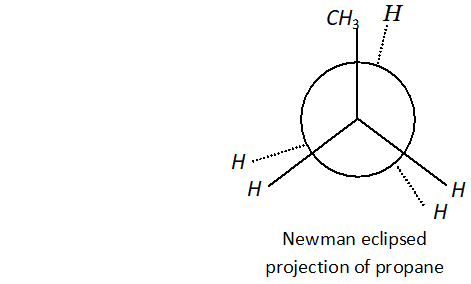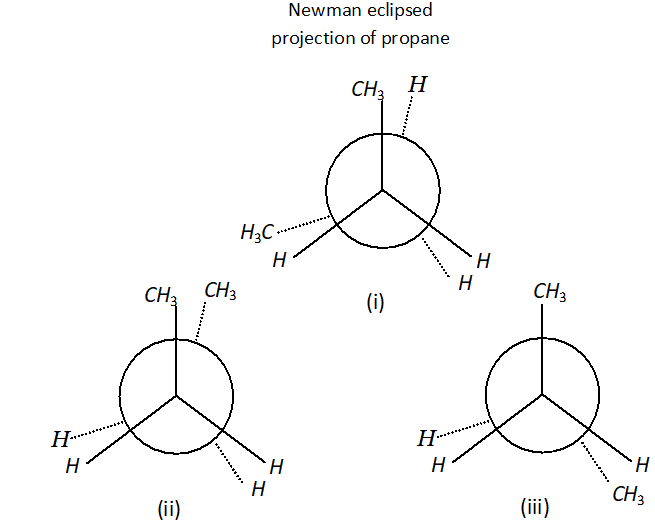(i), (ii) and (iii) Newman eclipsed projection of butane.
Q. Complete the reactions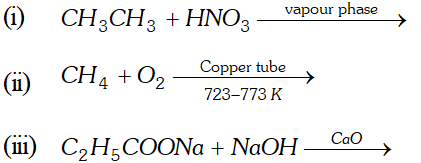Ans.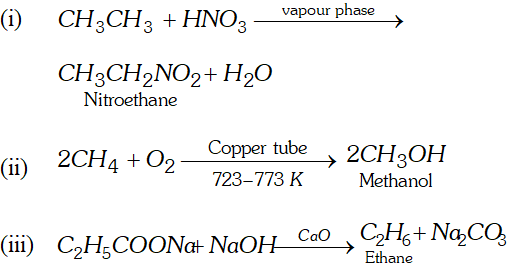Q. Write IUPAC names for the following molecules :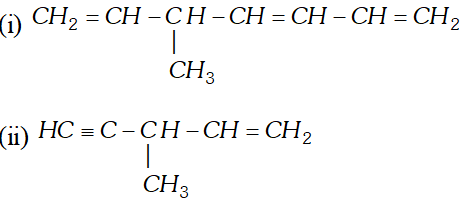[NCERT]
Ans. (i) 5 -methyl - hept $1,3,6$ -triene. (ii) 3 methyl pent $-1$ -ene- 4 -yne.
Q. Draw structure of the six isomeric pentenes, $C_{5} H_{10} .$ Specify as $E$ or $Z$ to each geometrical isomer. [NCERT]
Ans.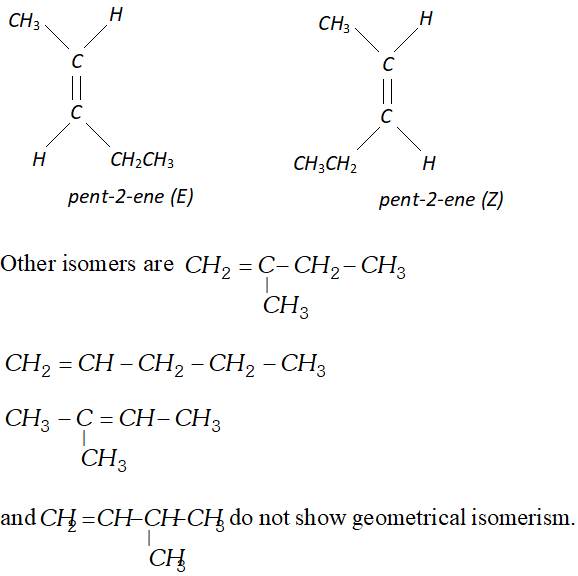Q. Convert ( i ) propene to 1 -bromopropane (ii) 2 -methyl propene to 2 -chloro $-2$ -methyl propane.
Ans.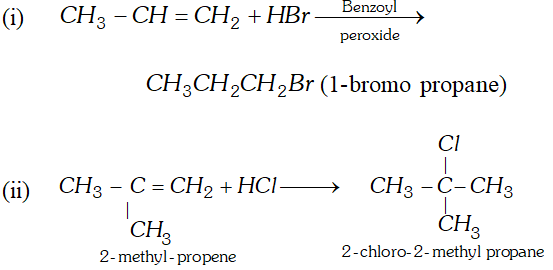Q. Why conjugated dienes undergo 1, 4-additions ? Explain.
Ans. Conjugated diene undergo 1, 4 addition due to more resonance stabilization of intermediate carbocation.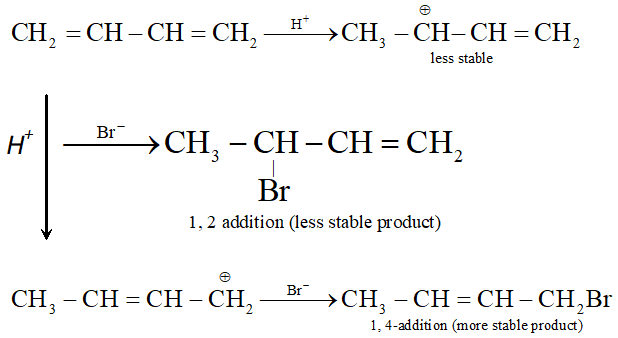Q. Which of the following polymerizes readily and why ? (i) Acetylene (ii) Ethene (iii) Buta-1, 3-diene. [NCERT]
Ans. (iii) Buta-1, 3-diene undergo polymerisation reaction readily because it will form resonance stabilized intermediate.
Q. What are the necessary conditions for any compound to show aromaticity ? [NCERT]
Ans. A compound is said to aromatic if there is delocalization of $\pi-$ electrons and it has planar structure. It should follow Huckel's Rule, i.e., in a conjugated, planar, cyclic system if number of delocalized electrons is $(4 n+2) \pi,^{c} n^{\prime}$ being integer, $i . e ., 1,2,3,$ etc. it will be aromatic, otherwise not, e.g. Benzene, naphthalene, anthracene have $6,10,14$ electrons in conjugated, planar, cyclic system.
Q. Write down the products of ozonolysis of $1,2$ -dimethyl benzene (o-xylene). How does the result support Kekule structure for benzene? [NCERT]
Ans.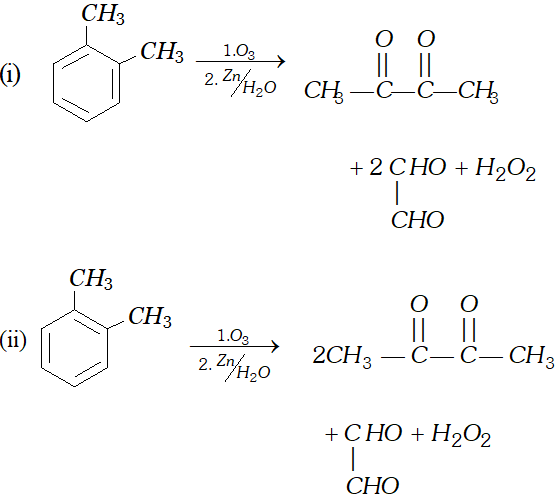These products prove the structure I and II (Kekule structures of benzene).
Q. Account for the following order of acidity, Acetylen e > Benzene> Hexane. [NCERT]
Ans. $-H-C \equiv C-H$ has $s p$ hybridised carbon which is most electronegative due to $50 \%$ s-character therefore it is most acidic. In Benzene, each carbon is $s p^{2}$ hybridised carbon $(33 \%$ of character), which is less electronegative than hybridised carbon, therefore, it is less acidic. In hexane, each $^{c} C^{\prime}$ is hybridised carbon $(25 \%$ s-character), which is least electronegative, therefore, it is least acidic.
Q. Why does benzene undergo electrophilic substitution reaction easily and nucleophilic substitution with difficulty ? [NCERT]
Ans. Benzene has $\pi-$ electrons, therefore, it can undergo electrophilic substitution reactions easily. Nucleophilic substitution are difficult because nucleophile will be repelled by $\pi$ -electrons.
Q. Write down the products and give mechanism of the following reactions :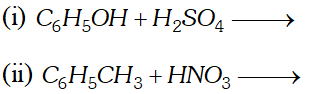[NCERT]
Ans.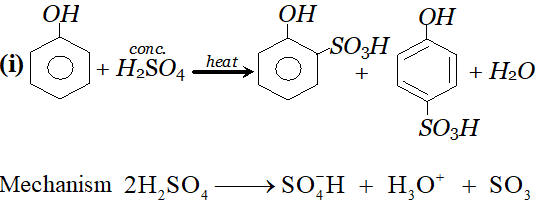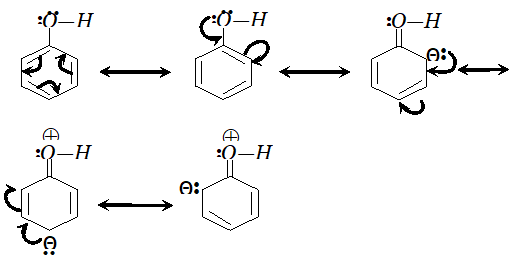since electron density is maximum at $o$ and $p$ -positions $S O_{3}$ will attack at $o$ and $p$ -positions.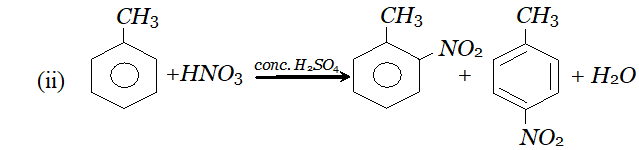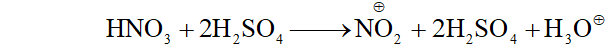$\mathrm{NO}_{2}$ will attack at $o$ and $p$ -positions because electron density is maximum at ortho and $p$ -positions.
Q. Arrange the following set of compounds in order of their decreasing reactivity with an electrophile, $E^{+}$ (i) Chlorobenzene, $2,4$ -dinitrochlorobenzene, p-nitrochlorobenzene (ii) Toluene, $p-H_{3} C-C_{6} H_{4}-C H_{3}, H_{3} C-C_{6} H_{4}-N O_{2}$ $p-O_{2} N-C_{6} H_{4}-N O_{2}$ [NCERT]
Ans.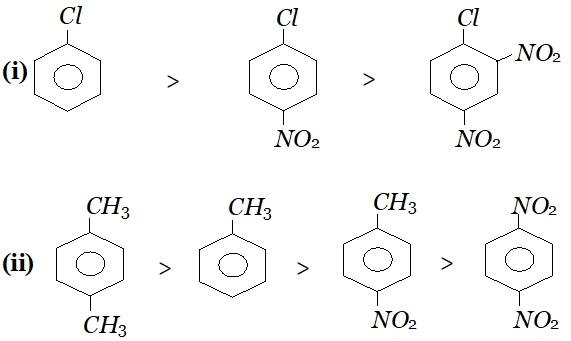Q. What happens when- (i) Sodium acetate is heated with soda lime. (ii) Acetylene is passed through ammoniacal silver nitrate solution. (iii) Chlorobenzene is condensed with chloral in the presence of sulphuric acid?
Ans. (i) When sodium acetate is heated with soda lime, methane gas will be formed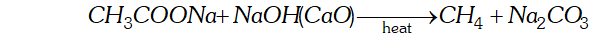(ii) When acetylene is passed through ammonical silver nitrate solution, white precipitate of silver acetylide will be formed.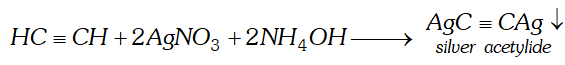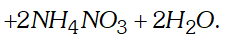(iii) When chlorobenzene is condensed with chloral, in presence of conc. $H_{2} S O_{4},$ DDT is formed.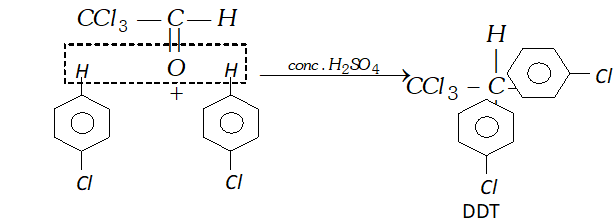Q. (i) Write a chemical equation for Friedel-Craft's reaction. (ii) Identify $A, B, C$ and $D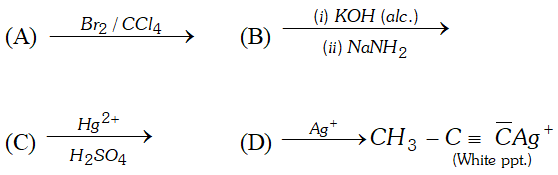$ in the following reactions:
Ans. (i) Friedel Crafts Reaction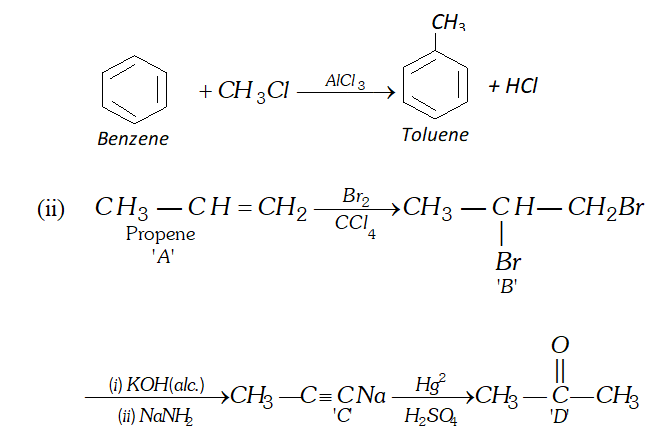Q. Bring out following conversions: (i) 2 -Bromo-propane to 1 Bromo-propane (ii) Methane to Ethane OR An unknown alkene $A$ on reductive ozonolysis gives two isomeric carbonyl compounds $B$ and $C$ of molecular formula $\mathrm{C}_{3} \mathrm{H}_{6} \mathrm{O}$. Write the structure of $A, B$ and $C$
Ans.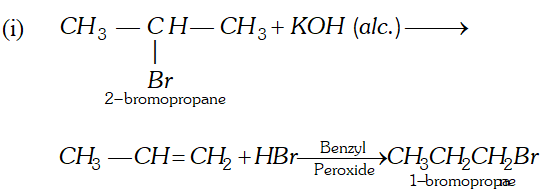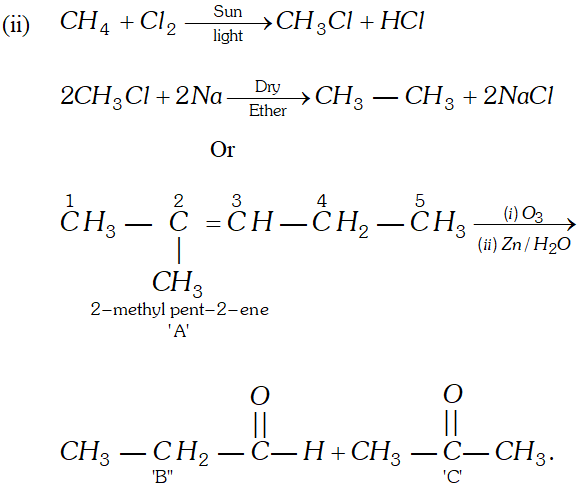Q. What is LPG ? State its composition, properties and applications. Or Give the full form of LPG and state its composition. Why it is considered a good fuel ?
Ans. Petroleum gas : It is a mixture of butane, propane, propene, isobutane and ethane. The main constituent of petroleum gas is butane. At ordinary pressure butane $\left(C_{4} H_{10}\right),$ Propane $\left(C_{3} H_{8}\right)$ and ethane $\left(C_{2} H_{6}\right)$ are gases. Under high pressure, they are easily liquefied. The petroleum gas which has been liquefied under pressure is called liquefied petroleum gas (LPG). The domestic gas cylinder contains LPG. LPG a better domestic fuel : Components of LPG burn easily and produce a lot of heat, so LPG is used as a good domestic fuel. Due to the following qualities, LPG is considered as a better fuel. (i) $\mathrm{LPG}$ is an efficient fuel as it has high calorific value, burning of 1 gram of LPG in air produces about 50 kilo joule of heat energy. (ii) LPG burns with a smokeless flame, so causes no pollution. (iii) It leaves no ash on burning so it is a clean fuel. (iv) On burning no poisonous gases are formed. (v) It is easy to handle and convenient in storing.
Q. Describe the process of fractional distillation of petroleum with the help of a labelled diagram of the distillation tower. Name any four/one products obtained. State the temperature at which each one of them is obtained.
Ans. Petroleum : It is a naturally occurring fossil fuel that is dark coloured, viscous and foul smelling oil. It is a mixture of several hydrocarbons and other elements such as sulphur, oxygen and nitrogen. Mostly it occurs deep down the earth between two nonporous rocks, that's why it is called as petroleum. (petra =rock, oleum $=$ oil ) or crude - oil.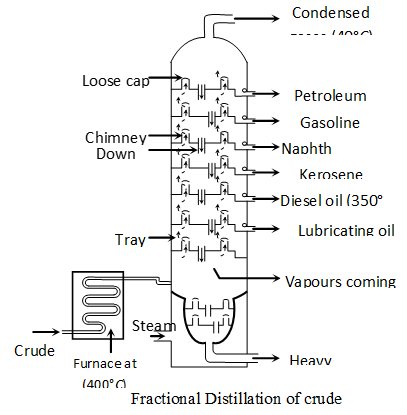Principle of refining of petroleum :”The process of separating different components of petroleum, is known as refining. It is carried out by the process of fractional distillation which is based on the fact that different components of petroleum have different boiling point ranges. The crude-oil is heated in a tank to a temperature of about and all the vapours formed are fed into a tall fractionating column near the bottom. Except asphalt, all the other components are in vapour state. As the mixture of hot vapours rises up in the column, it begins to cool The vapours of components having higher boiling point-range condense first in the lower part and vapours of components with lower boiling point-range condense later at the upper part of column. Therefore, the components with the highest boiling point condenses first and is collected in the lowest part of the column and the component having lowest boiling point condenses last and is collected in the top most part of column. The vapours that do not liquify, are taken out from the top of the column. This component is called petroleum-gas. The residual oil or liquid residue which does not vapourise in the column is called residual oil. It is collected in the base of column and subjected to further distillation. Products of petroleum : The various components obtained at different heights of fractional column, (in order of top to bottom of column) are as follows :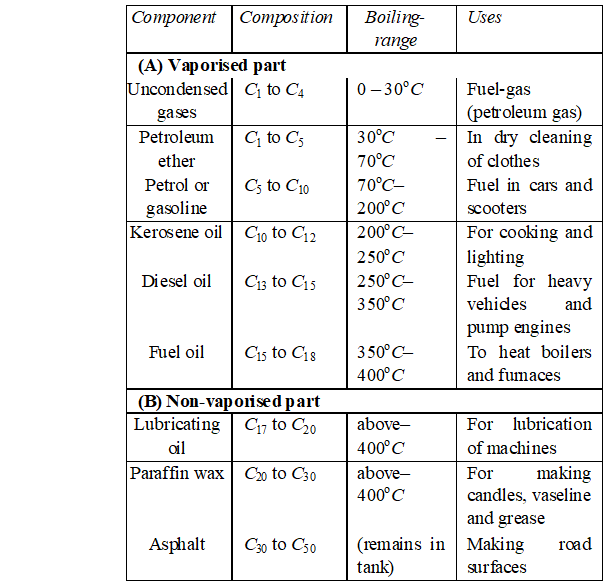Q. Give the products when the following reagents react with alkenes (i) Sulphuric acid (ii) halogens (iii) oxidation (iv) ozone.
Ans. (i) Reaction with sulphuric acid: Cold concentrated sulphuric -acid reacts with alkenes to gives addition products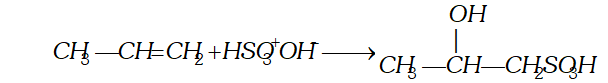(ii) Reaction with halogen : Halogens (chlorine and bromine only react at ordinary temperature and without exposure to $U V$ light to form vicinal halide.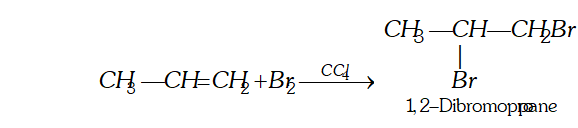(iii) Oxidation (a) Oxidation with dilute aqueous potassium permanganate solution : At low temperature alkenes form vicinal glycols.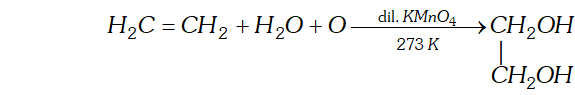[This reaction is called hydroxylation as hydroxyl groups are attached across the double bond. $]$ (b) With alkaline potassium permanganate at higher temperature : In such conditions cleavage of $C=C$ bond occurs forming carboxylic acids.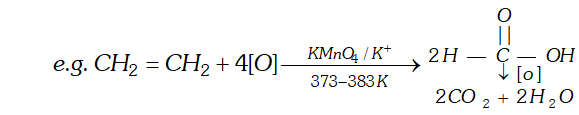(iv) Ozone: With ozone alkenes form ozonides which undergo cleavage to produce carbonyl compounds. This method was extensively used to determine the position of double bond in alkenes in past. Procedure : Ozone is passed through a solution of an alkene in inert solvent such as $\mathrm{CH}_{2} \mathrm{Cl}_{2}, \mathrm{CHCl}_{3}$ or $\mathrm{CCl}_{4}$ at temperature $196-200 K .$ Alkenes are oxidised to ozonised which are not usually isolated but reduced in situ, with zinc dust and water or $H_{2} / P d$ to give carbonyl compound. The location of double bond is assigned on the basis of products.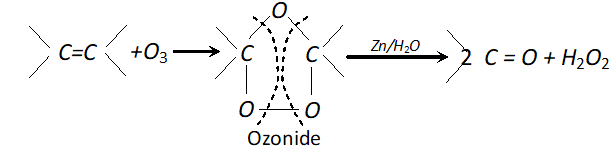(a) In case of symmetrical alkene, only one aldehyde is formed on reduction with zinc and water: $\mathrm{CH}_{3}-\mathrm{CH}=\mathrm{CH}-\mathrm{CH}_{3}+\mathrm{O}_{3} \longrightarrow$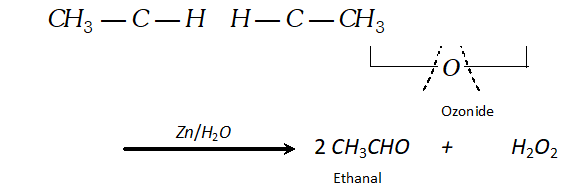(b) If double bond is terminal, methanal is one product and a higher aldehyde is other product. $\mathrm{CH}_{3}-\mathrm{CH}=\mathrm{CH}_{2}+\mathrm{O}_{3} \longrightarrow$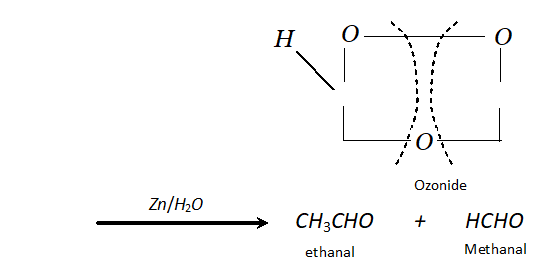(c) If ketone is formed, branching is there at C atom having double bond.Q. An alkene on ozonolysis gives 2 -butanone and 2 methylpropanal. What products will be obtained when it is treated with hot conc. $K M n O_{4} ?$
Ans. Let us first try to write the structure of alkene. The product of ozonolysis are :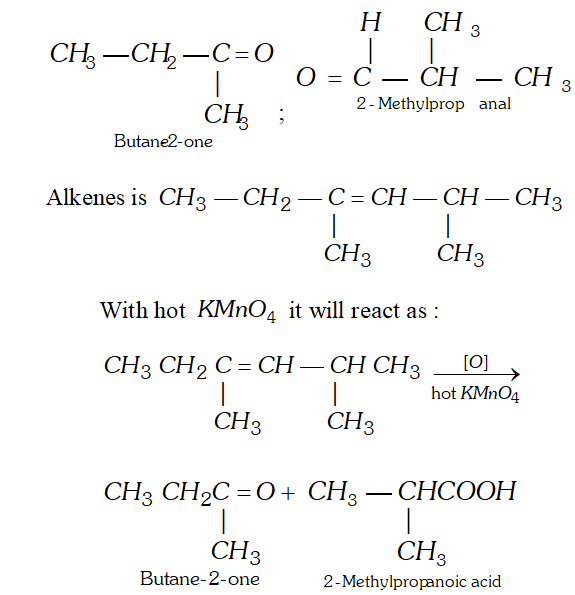Q. (i) What is Octane number? How does it atfect knocking? (ii) What type of isomerism is shown by methoxy methane and ethanol? (iii) Complete the following reactions: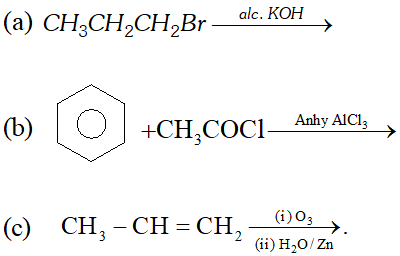Ans. (i) Octane number is defined as percentage of iso-octane in the mixture of iso-octane and $n$ -heptane which gives same knocking as sample fuel. Higher the octane number, lesser will be knocking. (ii) Functional isomerism.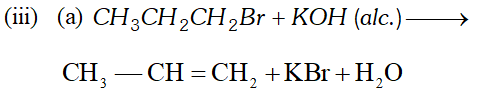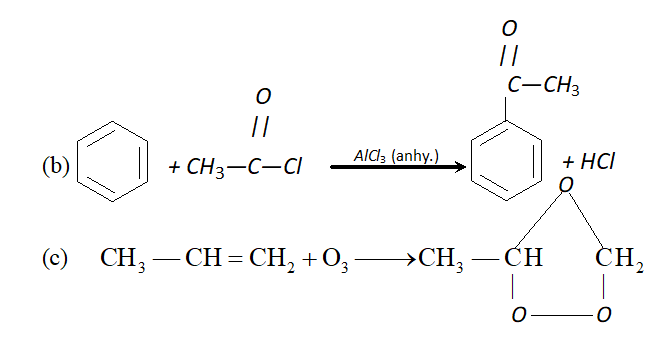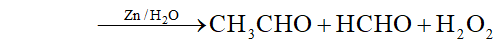Q. Complete the following reactions : (i) Isopropyl bromide $\frac{\text { alc. KOH }}{\text { Heat }} A \frac{H B r}{\text { Peroxide }} B$ (ii) $\quad n$ -Propyl alcohol $\frac{\text { Conc. } H_{2} S O_{4}}{443 K} A \frac{O_{2}, A g}{\text { Heat }}>B$ (iii) $1,1,2,2,$ -tetrachloroethane $\frac{z n, \text { alcohol }}{\text { Heat }}$ $A \frac{\text { Iron tube }}{675 \mathrm{K}}>B$ (iv) Acetylene $\frac{N a N H_{2}}{\longrightarrow} A \stackrel{C H_{3} C H_{2} B r}{\longrightarrow} B$ (v) Propyne $\frac{H_{2}, P d, B a S O_{4}}{\text { Quinoline }} \rightarrow A \frac{(i) O_{3}}{(i i) Z n, H_{2} O} B$
Ans.